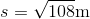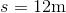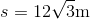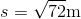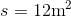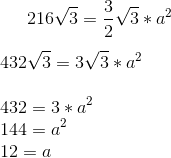## Example Questions

### Example Question #1 : How To Find The Length Of The Side Of A Hexagon

If the perimeter of a regular hexagon is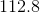, what is the length of a side of that regular hexagon?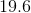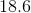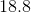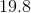Explanation:

Use the formula for perimeter to solve for the length of a side of the regular hexagon: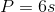Whereis perimeter andis the length of a side.

In this case: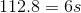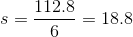### Example Question #1 : How To Find The Length Of The Side Of A Hexagon

Find the length of one side for a regular hexon with a perimeter of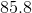.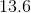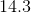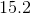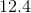Explanation:

Use the formula for perimeter to solve for the side length: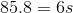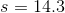### Example Question #2 : How To Find The Length Of The Side Of A Hexagon

Find the length of one side for a regular hexagon with a perimeter of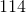.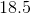Explanation:

Use the formula for perimeter to solve for the side length: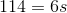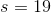### Example Question #3 : How To Find The Length Of The Side Of A Hexagon

What is the maximum length of each side for a regular hexago with a perimeter of?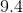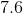8.8Explanation:

Use the formula for perimeter to solve for the side length: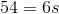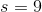### Example Question #1 : How To Find The Length Of The Side Of A Hexagon

What is the side of a Hexagon whose area is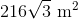?